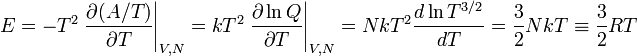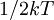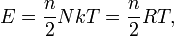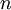# Ideal gas: Energy

(diff) ← Older revision | Latest revision (diff) | Newer revision → (diff)$E = -T^2 \left. \frac{\partial (A/T)}{\partial T} \right\vert_{V,N} = kT^2 \left. \frac{\partial \ln Q}{\partial T} \right\vert_{V,N}= NkT^2 \frac{d \ln T^{3/2}}{dT} = \frac{3}{2} NkT \equiv \frac{3}{2} RT$
where$R$ is the molar gas constant. This energy is all kinetic energy,$1/2 kT$ per degree of freedom, by equipartition. This is because there are no intermolecular forces, thus no potential energy. This result is valid only for a monoatomic ideal gas. The general expression would be$E = \frac{n}{2} NkT = \frac{n}{2} RT,$
where$n$ is the number of degrees of freedom. This number is 3 for atoms; if would be 6 in principle for diatomic molecules, but in normal conditions 5 is a very good approximation since vibrations are "frozen" (as explained in the entry about degrees of freedom.)